## 递归

• 递归就是在函数或过程里调用自身
• 在使用递归策略时，必须有一个明确的递归结束条件，称为递归出口

```local function fibo(n)
if n < 2 then return n end
return fibo(n-1) + fibo(n-2)
end

print(fibo(6))
```

## 辅助递归算法设计的工具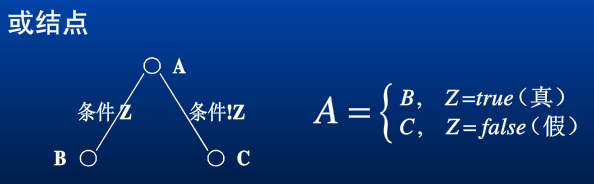A为或节点，A依不同条件会有两种不同的取值，B或C。或节点用空心圆表示。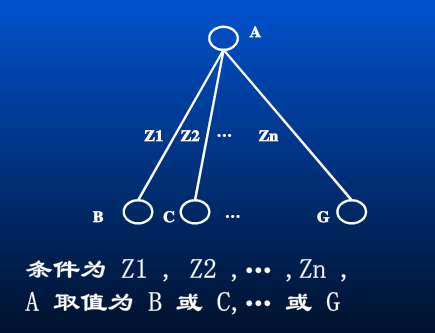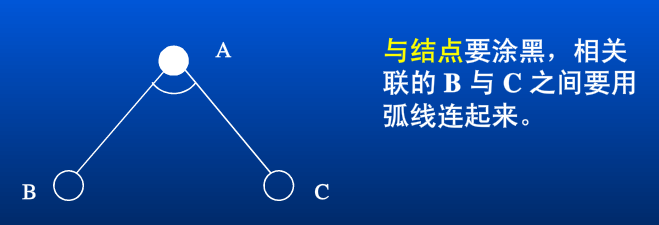A为与节点，A的最终取值是C节点的值，但为了求得C的值，得先求得B节点的值，C是B的函数。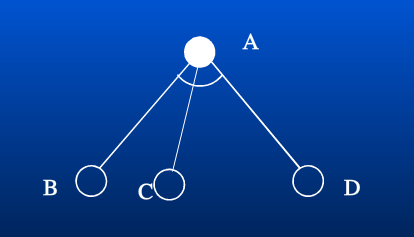A的最终取值是D节点的值，但是为了求得D的值，得先求B节点和C节点的值。从图上看得先求左边的节点的值，才能求最右边的节点的值。我们约定最右边D节点的值就是A节点的值。

## 什么样的程序是递归程序

```试编写一个函数fact(n)，求解n！。
n! = n * (n-1)!
```

Lua线性递归实现：

```local function fact(n)
return n == 1 and 1 or n * fact(n-1)
end

print(fact(3))
```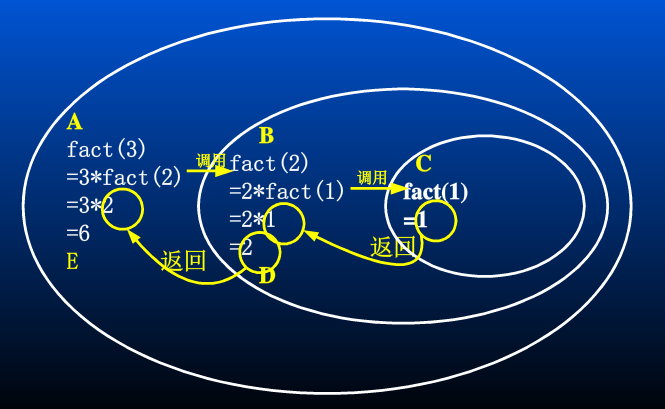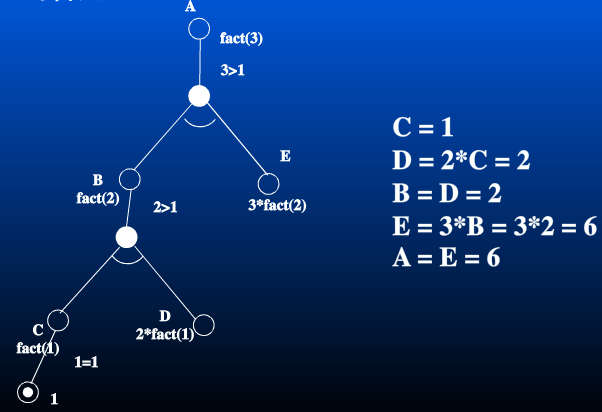fact(n)的与或图如下：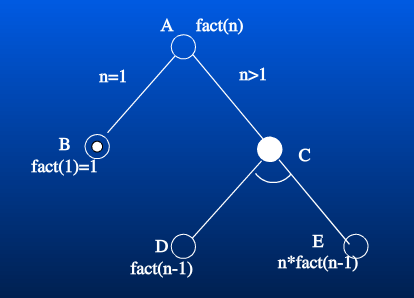A为或节点，B为直接可解节点，值为1。C为与节点，当n>1时，A的值即为C的值，而C的值即为E的值，为了求得E的值，需要先求出D的值，E是D的函数：E = D * n，也就是E的值等于D的值fact(n-1)乘以n。

## 递归算法举例 - 快速排序

```快速排序的基本思想：

```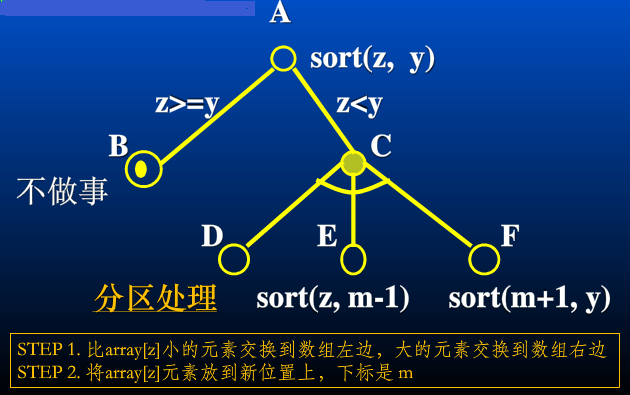A是或节点，当z >= y时，A的值是B节点的值，B是直接可解节点（递归边界）；当z < y时，A的值是C节点的值，C节点是与节点，C节点的值就是F节点的值，要想求得F，需要先求D、E的值，F是D、E的函数：sort(z, m-1);sort(m+1, y)。

```local function partition(arr, s, e)
local base, pos = arr[s], s
for ind=s+1, e do
if arr[ind] < base then
arr[pos] = arr[ind]
--move arr[pos+1 ... ind-1] -> arr[pos+2 ... ind]
local j = ind
while j > pos + 1 do
arr[j] = arr[j-1]
j = j - 1
end
pos = pos + 1
arr[pos] = base
end
end
return pos, arr
end

local function quicksort(arr, s, e)
local s, e = s or 1, e or #arr
if s >= e then return end
local pos = partition(arr, s, e)
quicksort(arr, s, pos-1)
quicksort(arr, pos+1, e)
end
```

## 递归算法举例-汉诺塔问题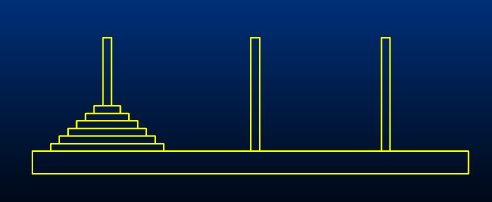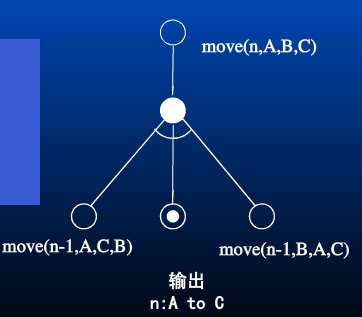```local function hanoi(n, A, B, C)
if n <= 0 then return end
hanoi(n-1, A, C, B)
print(string.format("move #%s from %s to %s.", n, A, C))
hanoi(n-1, B, A, C)
end
```

## 尾递归

• 什么是尾递归
当递归调用是整个函数体中最后执行的语句，并且它的返回值不属于表达式的一部分时，这个递归调用就是尾递归。
• 尾递归优化
当编译器检测到一个函数调用是尾递归的时候，它就覆盖当前的活动记录而不是在栈中去创建一个新的。编译器可以做到这点，因为递归调用是当前活跃期内最后一条待执行的语句，于是当这个调用返回时栈帧中并没有其他事情可做，因此也就没有保存栈帧的必要了。通过覆盖当前的栈帧而不是在其之上重新添加一个，这样所使用的栈空间就大大缩减了，这使得实际的运行效率会变得更高。
• 例子：
Lua尾递归实现求解n!:
```local function facttail(n, result)
return n == 1 and (result or 1) or facttail(n-1, n*(result or 1))
end
```
• 尾递归的优势：
尾递归是极其重要的，不用尾递归，函数的堆栈耗用难以估量，需要保存很多中间函数的堆栈。比如f(n, sum) = f(n-1) + value(n) + sum; 会保存n个函数调用堆栈，而使用尾递归f(n, sum) = f(n-1, sum+value(n)); 这样则只保留后一个函数堆栈即可，之前的可优化删去。
• 理解尾递归：
尾递归就是从最后开始计算，每递归一次就算出相应的结果，也就是说，函数调用出现在调用者函数的尾部，因为是尾部，所以根本没有必要去保存任何局部变量。直接让被调用的函数返回时越过调用者，返回到调用者的调用者去。
尾递归就是把当前的运算结果（或路径）放在参数里传给下层函数，深层函数所面对的不是越来越简单的问题，而是越来越复杂的问题——因为参数里带有前面若干步的运算路径。对于阶乘而言，越深并不意味着越复杂。
尾递归适用于运算对当前递归路径有依赖的问题，传统递归适用于运算对更深层递归有依赖的问题。